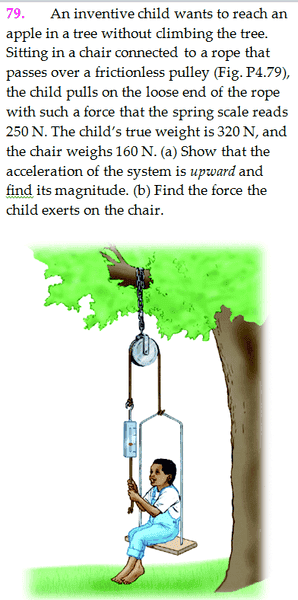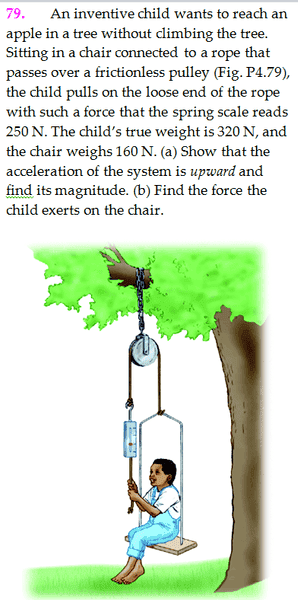# Child, Chair, and Spring Scale on Pulley: Forces

## Homework Statement## Homework Equations

$F=ma$

## The Attempt at a Solution

(a) Because the spring scale weighs 250 N, the effective weight on the child's side is 230 N. Therefore, $T-230 = \frac{230}{9.8} a$ and $T-250 = -\frac{250}{9.8} a$, so $a = 0.408$.

This is correct, but is the solution really legitimate? The "effective weight" argument seems a bit suspicious and nonrigorous.

(b) I have no idea where to even start. How would the "effective weights" work here? (Or would it even work?)

Thanks,
minimario

collinsmark
Homework Helper
Gold Member

## Homework Statement## Homework Equations

$F=ma$

## The Attempt at a Solution

(a) Because the spring scale weighs 250 N, the effective weight on the child's side is 230 N. Therefore, $T-230 = \frac{230}{9.8} a$ and $T-250 = -\frac{250}{9.8} a$, so $a = 0.408$.

This is correct, but is the solution really legitimate? The "effective weight" argument seems a bit suspicious and nonrigorous.

(b) I have no idea where to even start. How would the "effective weights" work here? (Or would it even work?)

Thanks,
minimario

Hello minimario,

Welcome to Physics Forums! :)

First, here's a link to get you started with using $\LaTeX$ on Phyisics Forums.

The "250 N" is not the weight of the spring scale, rather it is the reading on the spring scale. You should consider the spring scale itself to be massless. That it means is that the tension in the rope is 250 N.

Whenever you work with a massless and frictionless rope and pulley, it means that the tension of the rope on one side of the pulley is equal to the tension on the other side (I think it is valid from this problem to assume that the rope is also massless and the pully is both massless and frictionless).

(i) Draw a free body diagram (FBD) of all the forces acting on the child+chair combination. Don't forget that the rope has two ends! (Hint: you already know the tension on the rope. Just don't forget both ends.)

(ii) What is the net force acting on the child+chair combination (i.e., sum together all the force vectors, to find the net force vector [and don't forget they are vectors, not scalars])?

(iii) What is the the mass of the child+chair combination. (i.e., not the weight, but the mass)?

(iv) Invoke Newton's second law of motion to find the acceleration.

[Edit: For part b, start by drawing a FBD of all the forces acting only on the child. Hint: you know the child's mass (or you can calculate it now) and the child's acceleration from previous calculations. You also know the child's weight (gravitational force) and the force from the rope that the child is holding onto. Invoke Newton's second law again, and solve for the remaining force on the child from the chair.]

Last edited: